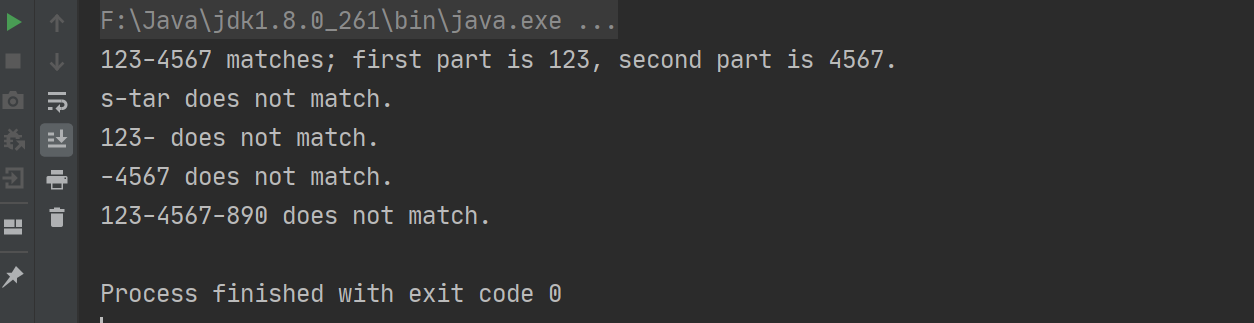# 在Java中，如何优雅地分割 String 字符串？## 使用方法

split 方法的一种声明为，

``public String[] split(String regex)``

``````String string = "86-15003455666";
String[] parts = string.split("-");
String part1 = parts; // 86
String part2 = parts; // 15003455666``````

split 方法的另一个声明为：

``public String[] split(String regex, int limit)``

regex 指的是 正则表达式分隔符，limit 指定的则是分割的份数，举个例子就明白了

``````String string = "004-556-42";
String[] parts = string.split("-", 2);   // 限定分割两份
String part1 = parts; // 004
String part2 = parts; // 556-42``````

``````String string = "86-15003455666";
String[] parts = string.split("(?<=-)");
String part1 = parts; // 86-
String part2 = parts; // 15003455666``````

``````String string = "86-15003455666";
String[] parts = string.split("(?=-)");
String part1 = parts; // 86
String part2 = parts; // -15003455666``````

## 妙用正则表达式

``````public class SplitExample {
//\d代表数字，+代表出现一次或多次。所以(\\d+)-(\\d+)匹配用"-"相连的两个数字串
// Pattern 对象是正则表达式的编译表示
private static Pattern twopart = Pattern.compile("(\\d+)-(\\d+)");

public static void checkString(String s)
{
// Matcher对象对输入字符串进行解释和匹配操作
Matcher m = twopart.matcher(s);
if (m.matches()) {
//m.group(1) 和 m.group(2) 存储分割后的子串
System.out.println(s + " matches; first part is " + m.group(1) +
", second part is " + m.group(2) + ".");
} else {
System.out.println(s + " does not match.");
}
}

public static void main(String[] args) {
checkString("123-4567");  // 匹配
checkString("s-tar");    // 字母序列，不匹配
checkString("123-");    // "-"右侧的数字串为空，不匹配
checkString("-4567");    // "-"左侧的数字串为空，不匹配
checkString("123-4567-890");    // 存在两个"-"，不匹配
}
}``````